﻿ 基于CUDA的多导体传输线方程迭代QR分解的并行算法

基于CUDA的多导体传输线方程迭代QR分解的并行算法Parallelization of QR Decomposition Algorithm in Multiconductor Transmission Line Equation Based on CUDA

Abstract: Based on the multi-conductor transmission line equation developed from the telegraph equation, as the number and complexity increase, the characteristic impedance matrix and conduction matrix and the dimension of the external excitation distribution source vector become large, resulting in the computational amount and memory overhead of solving the voltage or current on the cable composed of multi-conductor transmission lines. In this paper, we consider the general case of lossy conductor and lossy dielectric, and present the parallelization of iterative QR method for a matrix multiplied by the characteristic impedance matrix and admittance matrix based on CUDA. To prove the effectiveness of our method, we evaluate three different types of implementations: traditional single-thread C++, C++ accelerated with OpenMP and NvidiaCUDA. The evaluation result suggests that when the dimensions of problem reach hundreds, GPU implementation of CUDA is significantly more effective than CPU implementations of single-thread C++ and OpenMP.

1. 引言

2. N线多导体传输线方程的导出

$\frac{\text{d}}{\text{d}z}{i}_{n}\left(z,\omega \right)+{\sum }_{m=1}^{N}{{y}^{\prime }}_{nm}\left(\omega \right){v}_{m}\left(z,\omega \right)={i}_{n}^{\left(s\right)}\left(z,\omega \right),\text{\hspace{0.17em}}n=1,2,3,\cdots ,N$ (1)

$\frac{\text{d}}{\text{d}z}{v}_{n}\left(z,\omega \right)+{\sum }_{m=1}^{N}{{z}^{\prime }}_{nm}\left(\omega \right){i}_{m}\left(z,\omega \right)={v}_{n}^{\left(s\right)}\left(z,\omega \right),\text{\hspace{0.17em}}n=1,2,3,\cdots ,N$ (2)

$\frac{\text{d}}{\text{d}z}I\left(z,\omega \right)+{Y}^{\prime }\left(\omega \right)V\left(z,\omega \right)={I}^{\left(s\right)}\left(z,\omega \right)$ (3)

$\frac{\text{d}}{\text{d}z}V\left(z,\omega \right)+{Z}^{\prime }\left(\omega \right)I\left(z,\omega \right)={V}^{\left(s\right)}\left(z,\omega \right)$ (4)

$\begin{array}{l}\frac{\text{d}}{\text{d}z}\left[T\left(\omega \right)I\left(z,\omega \right)+V\left(z,\omega \right)\right]\\ =-\left[T\left(\omega \right){Y}^{\prime }\left(\omega \right)V\left(z,\omega \right)+{Z}^{\prime }\left(\omega \right)I\left(z,\omega \right)\right]+\left[T\left(\omega \right){I}^{\left(s\right)}\left(z,\omega \right)+{V}^{\left(s\right)}\left(z,\omega \right)\right]\end{array}$ (5)

${V}_{T}\left(z,\omega \right)=T\left(\omega \right)I\left(z,\omega \right)+V\left(z,\omega \right)$ (6)

${V}_{T}^{\left(s\right)}\left(z,\omega \right)=T\left(\omega \right){I}^{\left(s\right)}\left(z,\omega \right)+{V}^{\left(s\right)}\left(z,\omega \right)$ (7)

$\frac{\text{d}}{\text{d}z}{V}_{T}\left(z,\omega \right)=-\left[T\left(\omega \right){Y}^{\prime }\left(\omega \right)V\left(z,\omega \right)+{Z}^{\prime }\left(\omega \right)I\left(z,\omega \right)\right]+{V}_{T}^{\left(s\right)}\left(z,\omega \right)$ (8)

$T\left(\omega \right){Y}^{\prime }\left(\omega \right)V\left(z,\omega \right)+{Z}^{\prime }\left(\omega \right)I\left(z,\omega \right)=B\left(\omega \right){V}_{T}\left(z,\omega \right)$ (9)

$B\left(\omega \right)T\left(\omega \right)={Z}^{\prime }\left(\omega \right),\text{\hspace{0.17em}}\text{\hspace{0.17em}}B\left(\omega \right)=T\left(\omega \right){Y}^{\prime }\left(\omega \right)$ (10)

$T\left(\omega \right)=B\left(\omega \right){\left({Y}^{\prime }\left(\omega \right)\right)}^{-1}$ (11)

${B}^{2}\left(\omega \right)={Y}^{\prime }\left(\omega \right){Z}^{\prime }\left( \omega \right)$

${B}^{2}\left(\omega \right)={U}^{*}\left(\omega \right){Y}^{\prime }\left(\omega \right){Z}^{\prime }\left(\omega \right)U\left(\omega \right)=\text{diag}\left({\gamma }_{1}^{2},{\gamma }_{2}^{2},\cdots ,{\gamma }_{N}^{2}\right)$ (12)

${\gamma }_{k}=q{\left({\gamma }^{2}\right)}^{1/2},\text{\hspace{0.17em}}k=1,2,\cdots ,N$，则

${Y}^{\prime }\left(\omega \right){Z}^{\prime }\left(\omega \right)=U\left(\omega \right)={\left(U\left(\omega \right)q\text{diag}\left({\gamma }_{1},{\gamma }_{2},\cdots ,{\gamma }_{N}\right)\right)}^{*}\left(U\left(\omega \right)q\text{diag}\left({\gamma }_{1},{\gamma }_{2},\cdots ,{\gamma }_{N}\right)\right),q=±1$ (13)

${B}_{q}\left(\omega \right)=U\left(\omega \right)q\text{diag}\left({\gamma }_{1},{\gamma }_{2},\cdots ,{\gamma }_{N}\right),q=±1$ (14)

${T}_{q}\left(\omega \right)=U\left(\omega \right)q\text{diag}\left({\gamma }_{1},{\gamma }_{2},\cdots ,{\gamma }_{N}\right){\left[{Y}^{\prime }\left(\omega \right)\right]}^{-1},q=±1$ (15)

$\frac{\text{d}}{\text{d}z}{V}_{T,q}\left(z,\omega \right)=-{B}_{q}\left(\omega \right){V}_{T,q}\left(z,\omega \right)+{V}_{T,q}^{\left(s\right)}\left(z,\omega \right),q=±1$ (16)

${I}_{q}\left(z,\omega \right)={T}_{q}^{-1}\left(\omega \right)\left({V}_{T,q}\left(z,\omega \right)-{V}_{q}\left(z,\omega \right)\right)$ (17)

${V}_{q}\left(z,\omega \right)={Z}_{q}\left(\omega \right){I}_{q}\left(z,\omega \right)$ (18)

${Z}_{q}\left(\omega \right)={B}_{q}^{-1}\left(\omega \right){Z}^{\prime }\left(\omega \right)$ (19)

3. QR算法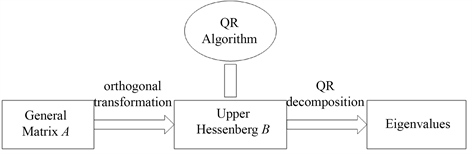Figure 1. Flow chart for QR algorithm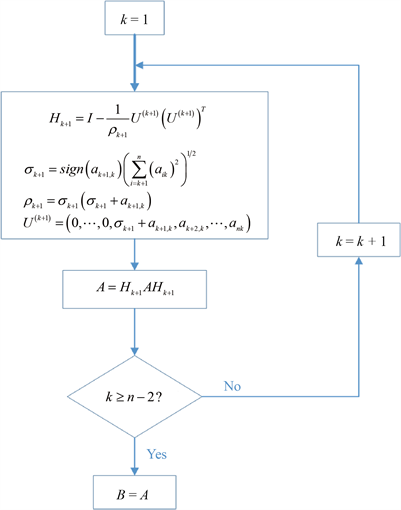Figure 2. The steps of turning a general matrix into a upper Hessenberg matrix

4. QR算法的并行化

QR算法如图4所示。其中第二部分，是QR分解，是迭代的，很难解耦。因此，我们选择第一部分来实现其并行性。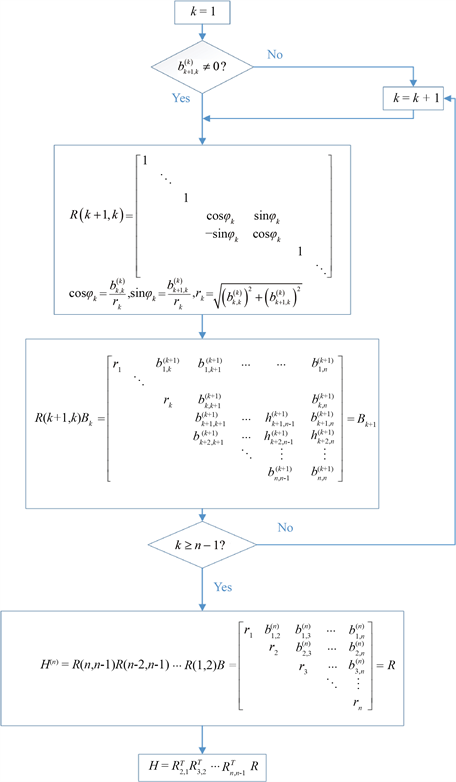Figure 3. The steps of QR decomposition over the upper Hessenberg matrix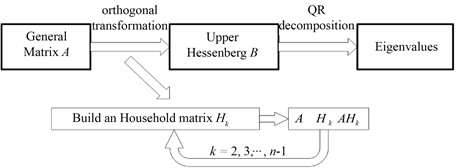Figure 4. Flow chart for QR algorithm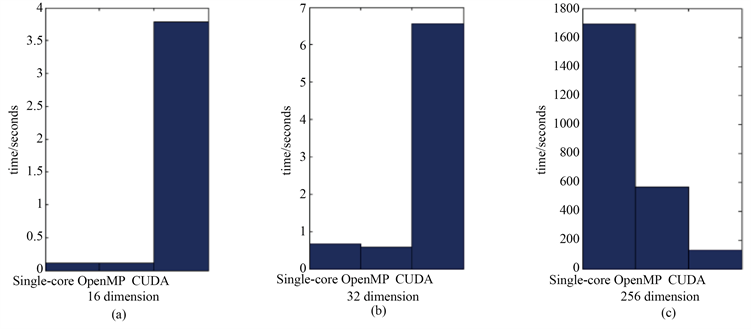Figure 5. Comparison of three algorithms in three dimensions

5. 试验结果Table 1. Running time of different algorithms

6. 结论

NOTES

*通讯作者。

 Baum, C.E., Liu, T.K. and Tesche, F.M. (1978) On the Analysis of General Multiconductor Transmission Line Networks. Kirtland AFB, Albuquerqut, NM, Interaction Note 350.

 Baum, C.E., Liu, T.K., Tesche, F.M. and Chang, S.K. (1977) Numerical Results for Multiconductor Transmission-Line Networks. Interaction Note 322, Air Force Weapons Laboratory, Albuquerque, NM, September 1977.

 Paul, D.R. (1994) Analysis of Multicomductor Transmission Lines. Wiley, New York.

 Tesche, F.M., Ianoz, M.V. and Karlsson, T. (1997) EMC Analysis Methods and Computational Models. Wiley, New York.

 Tesche, F.M., Keen, J. and Butler, C.M. (2004) Example of the Use of the BLT Equation for EM Field Propagation and Coupling Calculations. Kirtland AFB. Albuquerque, NM, Interaction Note 591, 16 August 2004.

 Paletta, L., Parmantier, J.-P., Issac, F., Dumtas, P. and Alliot, J.-C. (2002) Susceptibility Analysis of Wiring in a Complex System Combining a 3-D Solver and a Transmission-Line Network Simulation. IEEE Transactions on EMC, 44, 309-317.
https://doi.org/10.1109/TEMC.2002.1003395

 Kincaid, D.R. and Cheney, E.W. (2001) Numerical Analysis: Mathematics of Scientific Computing. American Mathematical Society, Providence.

 Shane Cook. CUDA并行程序设计: GPU编程指南[M]. 苏统华, 李东, 李松泽, 译. 北京: 机械工业出版社, 2014.

Top